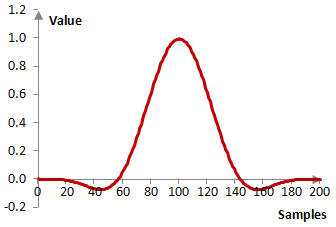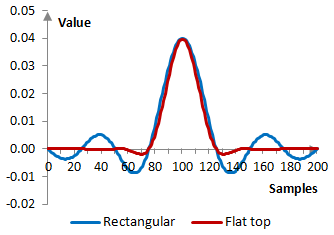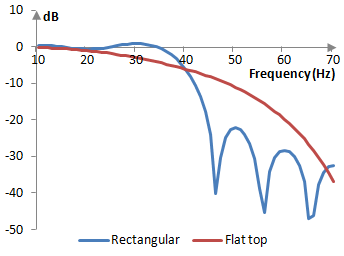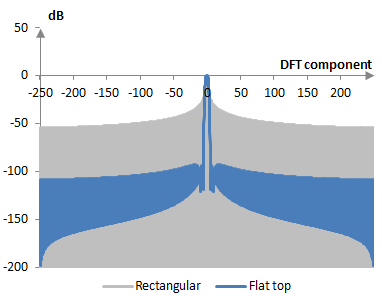# Flat top window

The flat top window coefficients are given by the following formula

$$a(k)= 0.21557895-0.41663158 \, \cos(\frac{2 \pi k}{N-1})+0.277263158 \, \cos(\frac{4 \pi k}{N-1})$$ $$- 0.083578947 \, \cos(\frac{6 \pi k}{N-1}) + 0.006947368 \, \cos(\frac{8 \pi k}{N-1})$$

where N is the length of the filter and k = 0, 1, …, N – 1.

## An example flat top window

Consider a finite impulse response (FIR) low pass filter of length N = 201. The following is the flat top window.Given a sampling frequency of 2000 Hz and a filter cutoff frequency of 40 Hz, the impulse response of the filter with a rectangular window (with no window) and with the flat top window is as follows.The magnitude response of the same filter is shown on the graph below.## Measures for the flat top window

The following graph shows the discrete Fourier transform of the flat top window against the transform of the rectangular window.The following are selected measures for the flat top window.

 Coherent gain 0.22 Equivalent noise bandwidth 3.78 Processing gain -5.77 dB Scalloping loss -0.01 dB Worst case processing loss -5.78 dB Highest sidelobe level -93.6 dB Sidelobe falloff -12.1 dB / octave, -40.1 dB / decade Main lobe is -3 dB 3.72 bins Main lobe is -6 dB 4.00 bins Overlap correlation at 50% overlap -0.015 Amplitude flatness at 50% overlap -0.110 Overlap correlation at 75% overlap 0.044 Amplitude flatness at 75% overlap 0.938

Window

### Filtered HTML

• Freelinking helps you easily create HTML links. Links take the form of [[indicator:target|Title]]. By default (no indicator): Click to view a local node.